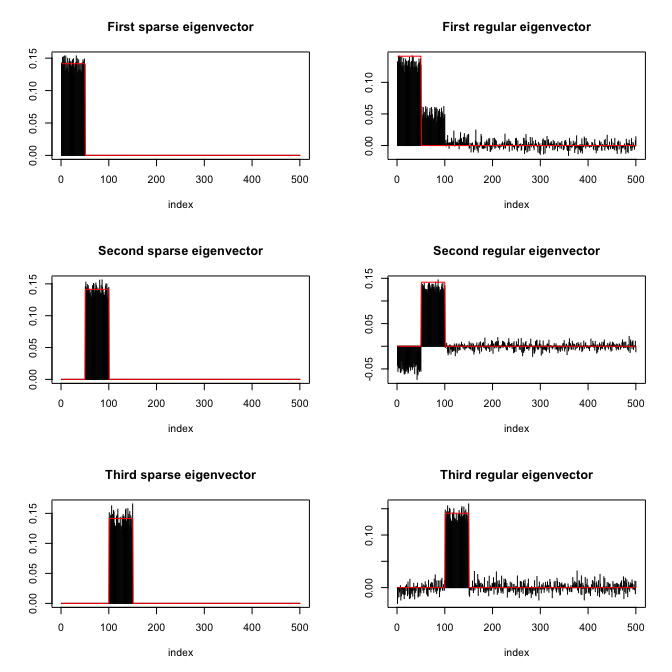Computation of Sparse Eigenvectors of a Matrix

Computation of sparse eigenvectors of a matrix (aka sparse PCA) with running time 2-3 orders of magnitude lower than existing methods and better final performance in terms of recovery of sparsity pattern and estimation of numerical values. Can handle covariance matrices as well as data matrices with real or complex-valued entries. Different levels of sparsity can be specified for each individual ordered eigenvector and the method is robust in parameter selection. See vignette for a detailed documentation and comparison, with several illustrative examples. The package is based on the paper: K. Benidis, Y. Sun, P. Babu, and D. P. Palomar (2016). "Orthogonal Sparse PCA and Covariance Estimation via Procrustes Reformulation," IEEE Transactions on Signal Processing .

This package provides functions to compute sparse eigenvectors (while keeping their orthogonality) or sparse PCA from either the covariance matrix or directly the data matrix.

Usage of spEigen()

We start by loading the package and generating synthetic data with sparse eigenvectors:

Then, we estimate the covariance matrix with cov(X) and compute its sparse eigenvectors:

We can assess how good the estimated eigenvectors are by computing the inner product with the original eigenvectors (the closer to 1 the better):

Finally, the following plot shows the sparsity pattern of the eigenvectors (sparse computation vs. classical computation):Usage of spEigenCov()

The function spEigenCov() requires more samples than the dimension (otherwise some regularization is required). Therefore, we generate data as previously with the only difference that we set the number of samples to be n=600.

Then, we compute the covariance matrix through the joint estimation of sparse eigenvectors and eigenvalues:

Again, we can assess how good the estimated eigenvectors are by computing the inner product with the original eigenvectors:

Finally, we can compute the error of the estimated covariance matrix (sparse eigenvector computation vs. classical computation):

sparseEigen 0.1.0

• Initial release.

Reference manual

install.packages("sparseEigen")

0.1.0 by Daniel P. Palomar, 4 years ago

https://github.com/dppalomar/sparseEigen, https://www.danielppalomar.com, https://doi.org/10.1109/TSP.2016.2605073

Report a bug at https://github.com/dppalomar/sparseEigen/issues

Browse source code at https://github.com/cran/sparseEigen

Authors: Konstantinos Benidis [aut] , Daniel P. Palomar [cre, aut]

Documentation:   PDF Manual